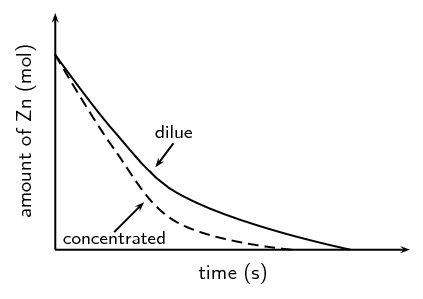We think you are located in United States. Is this correct?

# End of chapter exercises

Textbook Exercise 7.5

Explain the following:

Reaction rate

The reaction rate describes how quickly reactants are used up or how quickly products are formed.

Collision theory

Reactant particles must collide with the correct energy and orientation for the reactants to change into products.

For a reaction to occur the reactant particles must:

• collide

• have enough energy

• have the right orientation at the moment of impact

Activation energy

The minimum energy required for a chemical reaction to proceed.

Catalyst

A catalyst speeds up a chemical reaction. It increases the reaction rate by lowering the activation energy for a reaction and remains unchanged after the reaction is complete.

$$2\text{Mg}(\text{s}) + \text{O}_{2}(\text{g})$$ $$\to$$ $$2\text{MgO}(\text{s})$$

$$\text{1}$$ $$\text{g}$$ of $$\text{Mg}$$ is oxidised. The reaction takes 1 day to reach completion. Which is the correct reaction rate for the oxidation of $$\text{Mg}$$?

1. $$\text{2,87} \times \text{10}^{-\text{7}}$$ $$\text{mol·s^{-1}}$$

2. $$\text{1,74} \times \text{10}^{-\text{3}}$$ $$\text{mol·s^{-1}}$$

3. $$\text{4,76} \times \text{10}^{-\text{7}}$$ $$\text{mol·s^{-1}}$$

4. $$\text{3,62} \times \text{10}^{-\text{7}}$$ $$\text{mol·s^{-1}}$$

M(Mg) = $$\text{24,3}$$ $$\text{g·mol^{-1}}$$

n = $$\dfrac{\text{m (g)}}{\text{M (g·mol}^{-1}{\text{)}}} = \dfrac{\text{1} \text{ g}}{\text{24,3} {\text{ g·mol}}^{-1}}$$ = $$\text{0,0412}$$ $$\text{mol}$$

time = $$\text{1}$$ $$\text{day}$$ $$\times \dfrac{\text{24} {\text{ hours}}}{\text{1} {\text{ day}}}$$ = $$\text{24}$$ $$\text{hours}$$ $$\times \dfrac{\text{60} {\text{ minutes}}}{\text{1} {\text{ hour}}}$$ = $$\text{1,44} \times \text{10}^{\text{3}}$$ $$\text{minutes}$$ $$\times \dfrac{\text{60} {\text{ seconds}}}{\text{1} {\text{ minute}}}$$ = $$\text{8,64} \times \text{10}^{\text{4}}$$ $$\text{s}$$

reaction rate = $$\dfrac{\text{n (mol)}}{\text{t (s)}} = \dfrac{\text{0,0412} {\text{ mol}}}{\text{8,64} \times \text{10}^{\text{4}} {\text{ s}}}$$ = c) $$\text{4,76} \times \text{10}^{-\text{7}}$$ $$\text{mol·s^{-1}}$$

$$\text{10}$$ $$\text{g}$$ of magnesium ribbon reacts with a $$\text{0,15}$$ $$\text{mol·dm^{-3}}$$ solution of hydrochloric acid at a temperature of $$\text{25}$$ $$\text{℃}$$.

Write a balanced chemical equation for the reaction.

$$\text{Mg}(\text{s}) + 2\text{HCl}(\text{aq})$$ $$\to$$ $$\text{MgCl}_{2}(\text{aq}) + \text{H}_{2}(\text{g})$$

State two ways of increasing the average rate of production of $$\text{H}_{2}$$ (g).

Cut the magnesium into small pieces, increase the concentration of hydrochloric acid.

A table of the results is given below:

 Time elapsed (min) Vol of $$\text{H}_{2}$$(g) ($$\text{cm^{3}}$$) $$\text{0}$$ $$\text{0}$$ $$\text{0,5}$$ $$\text{17}$$ $$\text{1,0}$$ $$\text{25}$$ $$\text{1,5}$$ $$\text{30}$$ $$\text{2,0}$$ $$\text{33}$$ $$\text{2,5}$$ $$\text{35}$$ $$\text{3,0}$$ $$\text{35}$$

Plot a graph of volume versus time for these results.

Remember to convert time to seconds and volume to $$\text{dm^{3}}$$: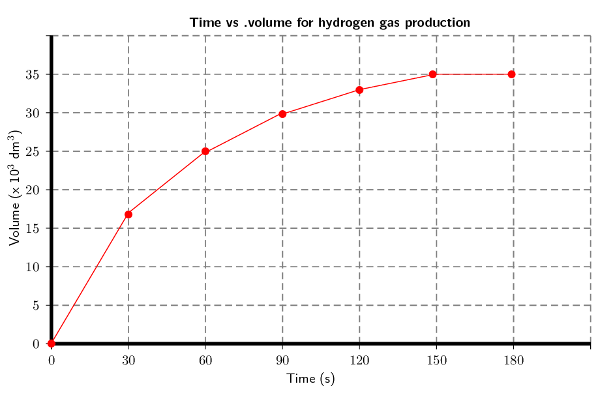Explain the shape of the graph during the following two time intervals: t = $$\text{0}$$ to t = $$\text{120}$$ $$\text{s}$$ and then t = $$\text{150}$$ and t = $$\text{180}$$ $$\text{s}$$ by referring to the volume of $$\text{H}_{2}$$ (g) produced.

(IEB Paper 2, 2001)

There is a sharp increase in the volume of hydrogen gas produced for the first 120 seconds. This is when the reaction is starting and there are lots of reactants. Between 150 and 180 seconds equilibrium is established and so the volume of hydrogen gas that has been produced remains constant.

Consider the following reaction, which takes place in a closed container:

$$\text{A}(\text{s}) + \text{B}(\text{g})$$ $$\to$$ $$\text{AB}(\text{g})$$

If you wanted to increase the average rate of the reaction, which of the following would you do?

1. decrease the concentration of B

2. grind A into a fine powder

3. decrease the pressure

(IEB Paper 2, 2002)

b) grind A to a fine powder

Consider the following reaction and calculate the reaction rates for the situations that follow:

$$2\text{N}_{2}\text{O}_{5}(\text{g})$$ $$\to$$ $$4\text{NO}_{2}(\text{g}) + \text{O}_{2}(\text{g})$$

$$\text{1,35}$$ $$\text{mol}$$ $$\text{N}_{2}\text{O}_{5}$$ is used and the reaction goes to completion in 30 minutes.

time = $$\text{30}$$ $$\text{minutes}$$ $$\times \dfrac{\text{60} {\text{ s}}}{\text{1} {\text{ minute}}}$$ = $$\text{1 800}$$ $$\text{s}$$

reaction rate of $$\text{N}_{2}\text{O}_{5}$$ used is = $$=\dfrac{\text{1,35}\text{ mol}}{\text{1 800}\text{ s}}=$$ $$\text{7,5} \times \text{10}^{-\text{4}}$$ $$\text{mol·s^{-1}}$$

$$\text{2,7}$$ $$\text{mol}$$ $$\text{N}_{2}\text{O}_{5}$$ is used instead and the reaction goes to completion in 60 minutes.

time = $$\text{60}$$ $$\text{minutes}$$ $$\times \dfrac{\text{60} {\text{ s}}}{\text{1} {\text{ minute}}}$$ = $$\text{3 600}$$ $$\text{s}$$

reaction rate of $$\text{N}_{2}\text{O}_{5}$$ used is = $$=\dfrac{\text{2,7}\text{ mol}}{\text{3 600}\text{ s}}=$$ $$\text{7,5} \times \text{10}^{-\text{4}}$$ $$\text{mol·s^{-1}}$$

$$\text{CaCO}_{3}(\text{s}) + 2\text{HCl}(\text{aq})$$ $$\to$$ $$4\text{NO}_{2}(\text{g}) + \text{O}_{2}(\text{g})$$

$$\text{2}$$ $$\text{g}$$ of $$\text{CaCO}_{3}$$ is placed in excess $$\text{HCl}$$. The reaction goes to completion over 4 hours.

M($$\text{CaCO}_{3}$$) = ($$\text{40,1}$$ + $$\text{12}$$ + $$\text{3}$$ x $$\text{16}$$) $$\text{g·mol^{-1}}$$ = $$\text{100,1}$$ $$\text{g·mol^{-1}}$$

$$n=\dfrac{m}{M}=\dfrac{2 {\text{ g}}}{\text{100,1}\text{ g·mol^{-1}}}=\text{0,02}\text{ mol}$$

time = $$\text{4}$$ $$\text{hours}$$ $$\times \dfrac{\text{60} {\text{ minutes}}}{\text{1} {\text{ hour}}}$$ = $$\text{240}$$ $$\text{minutes}$$ $$\times \dfrac{\text{60} {\text{ s}}}{\text{1} {\text{ minute}}}$$ = $$\text{14 400}$$ $$\text{s}$$

reaction rate $$\text{CaCO}_{3}$$ used is = $$=\dfrac{\text{0,02}\text{ mol}}{\text{14 400}\text{ s}}=$$ $$\text{1,39} \times \text{10}^{-\text{6}}$$ $$\text{mol·s^{-1}}$$

The concentration of the $$\text{HCl}$$ is increased and so the reaction takes $$\text{2,5}$$ $$\text{hours}$$ instead.

M($$\text{CaCO}_{3}$$) = $$\text{100,1}$$ $$\text{g·mol^{-1}}$$

$$n=\dfrac{m}{M}=\text{0,02}\text{ mol}$$

time = $$\text{2,5}$$ $$\text{hours}$$ $$\times \dfrac{\text{60} {\text{ minutes}}}{\text{1} {\text{ hour}}}$$ = $$\text{150}$$ $$\text{minutes}$$ $$\times \dfrac{\text{60} {\text{ s}}}{\text{1} {\text{ minute}}}$$ = $$\text{9 000}$$ $$\text{s}$$

reaction rate $$\text{CaCO}_{3}$$ used is = $$\dfrac{\text{0,02}\text{ mol}}{\text{9 000}\text{ s}}=$$ $$\text{2,22} \times \text{10}^{-\text{6}}$$ $$\text{mol·s^{-1}}$$

$$\text{3,3}$$ $$\text{g}$$ of $$\text{CaCO}_{3}$$ is used with a dilute $$\text{HCl}$$. $$\text{0,6}$$ $$\text{g}$$ of solid $$\text{CaCO}_{3}$$ remains after $$\text{4,7}$$ hours.

M($$\text{CaCO}_{3}$$) = $$\text{100,1}$$ $$\text{g·mol^{-1}}$$

m of $$\text{CaCO}_{3}$$ used during the reaction = $$\text{3,3}$$ - $$\text{0,6}$$ = $$\text{2,7}$$ $$\text{g}$$

$$n=\dfrac{m}{M}=\dfrac{\text{2,7}\text{ g}}{\text{100,1}\text{ g·mol^{-1}}}=\text{0,027}\text{ mol}$$

time = $$\text{4,7}$$ $$\text{hours}$$ $$\times \dfrac{\text{60} {\text{ minutes}}}{\text{1} {\text{ hour}}}$$ = $$\text{282}$$ $$\text{minutes}$$ $$\times \dfrac{\text{60} {\text{ s}}}{\text{1} {\text{ minute}}}$$ = $$\text{16 920}$$ $$\text{s}$$

reaction rate = $$\dfrac{\text{0,027}\text{ mol}}{\text{16 920}\text{ s}}=$$ $$\text{1,6} \times \text{10}^{-\text{6}}$$ $$\text{mol·s^{-1}}$$

Given: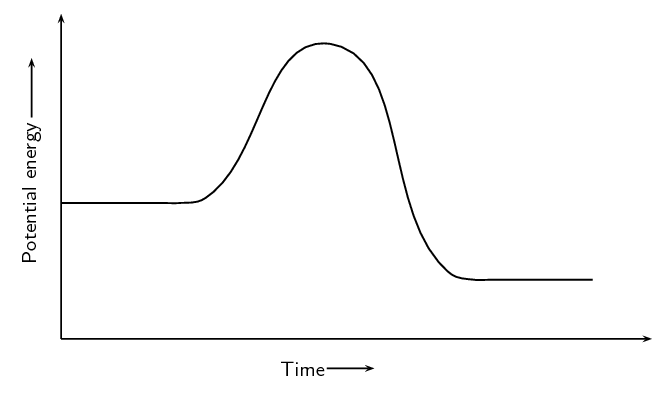A catalyst is added to this reaction. On the same set of axes draw the graph to represent this.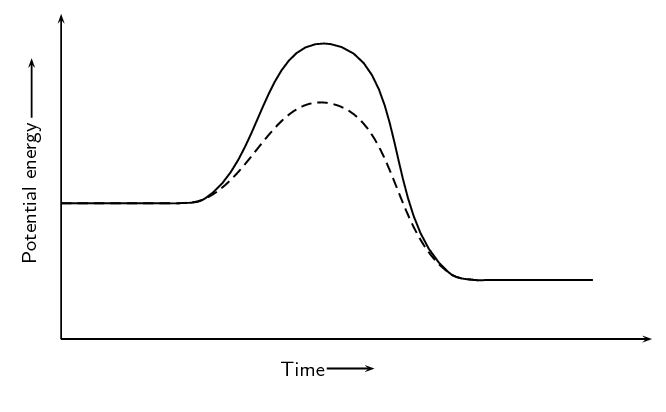A group of learners use the reaction between zinc and sulfuric acid to investigate one of the factors that affects reaction rate. The equation below represents the reaction that takes place.

$$\text{Zn}(\text{s}) + \text{H}_{2}\text{SO}_{4}(\text{aq})$$ $$\to$$ $$\text{ZnSO}_{4}(\text{aq}) + \text{H}_{2}(\text{g})$$

They add $$\text{6,5}$$ $$\text{g}$$ of zinc granules to excess dilute sulfuric acid and measure the mass of zinc used per unit time.

The learners then repeat the experiment using excess concentrated sulfuric acid.

The results obtained for the reaction using dilute sulfuric acid are represented in the graph below: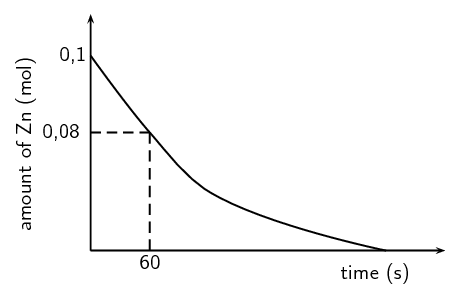Define the term reaction rate

Amount of reactants used per unit time or amount of products formed per unit time.

Give a reason why the acid must be in excess

To ensure that (nearly) all zinc is used up or to ensure that zinc is a limiting reagent (and the acid is not a limiting reagent).

Write down a hypothesis for this investigation.

(Learners must correctly identify the dependent and independent variables. They must predict the correct relationship between these variables)

Reaction rate increases with increase in concentration.

Reaction rate decreases with decrease in concentration.

Reaction rate is directly proportional to concentration.

The higher the concentration the faster the average rate of the reaction.

Give a reason why the learners must use the same amount of zinc granules in both experiments.

To make it a fair test.

or

Ensure validity (reliability) of results.

or

So that the contact/surface area may not influence the reaction rate. The surface area must not change.

or

It is the controlled variable.

or

To ensure there is only one independent variable.

Using the graph, calculate the mass of zinc used from t = 0 s to t = 60 s.

Number of moles used = $$\text{0,1}$$ - $$\text{0,08}$$ = $$\text{0,02}$$ $$\text{mol}$$

M(Zn) = $$\text{65,4}$$ $$\text{g}$$

$$\text{n}=\dfrac{\text{m}}{\text{M}}$$, therefore m = n x M

m = $$\text{0,02}$$ $$\text{mol}$$ x $$\text{65,4}$$ $$\text{gmol^{-1}}$$ = $$\text{1,31}$$ $$\text{g}$$

Calculate the average rate of the reaction (in grams per second) during the first 60 s.

Average rate = $$\dfrac{\text{mass Zn used (g)}}{\text{time (s)}} = \dfrac{\text{1,31}\text{ g}}{\text{60}\text{ s}}$$

= $$\text{0,022}$$ $$\text{g.s^{-1}}$$

Copy the above graph and on the same set of axes use a dotted line to show the curve that will be obtained when concentrated sulfuric acid is used. No numerical values are required.

(NSC, Paper 2, 2011)

Criteria for graph - Concentrated sulfuric graph has steeper slope than original graph and intercepts with x-axis earlier.